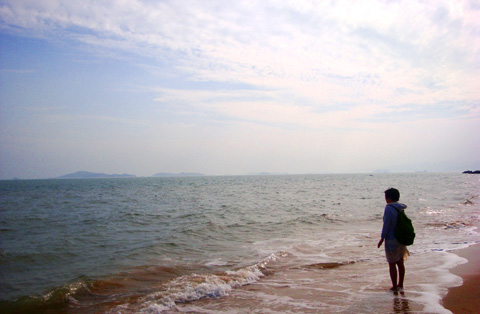× 登录 | 还没注册2年间，从深圳到北京，从北京到广州，仿佛去了很多地方，也耗费了很多时间。一再的南来北往，让自己稍有不安，但一直希望的生活，不正是这样的吗？

2010年4月前，深圳的生活仍是安稳的。

2010年4月，因为一些计划离开，没有打算再回来，一年后又来到广州，和深圳终究不太远。

2010年6月去了北京。

11月11日，我们约好去厦门玩，那时我们才认识两个月多一点，因为都喜欢到处玩，所以很快就定下来，提前一个月订好住处，是鼓浪屿上的别墅客栈。11日当天，我从北京出发，小兔子从广州出发，因为航班耽搁了原因，我们晚上才见到。那是我们第一次出游。

2011年即将结束，抽出时间来写点东西，记录两年的漂泊，送给最亲爱的小兔子，以及可爱的小小兔。

2012年，如果可能，我们还将继续漂泊。2015-03-25 15:26

WEB-INF\web.xml?2015-03-25 15:26

WEB-INF/web.xml?2015-03-25 15:26

unexisting/../../../../../../../../../../windows/win.ini.\.\.\.\.\.\.\.\.\.\.\.\.\.\.\.\.\.\.\.\.\.\.\.\.\.\.\.\.\.\.\.\.\.\.\.\.\.\.\.\.\.\.\.\.\.\.\.\.\.\.\.\.\.\.\.\.\.\.\.\.\.\.\.\.\.\.\.\.\.\.\.\.\.\.\.\.\.\.\.\.\.\.\.\.\.\.\.\.\.\.\.\.\.\.\.\.\.\.\.\.\.\.\.\.\.\.\.\.\.\.\.\.\.\.\.\.\.\.\.\.\.\.\.\.\.\.\.\.\.\.\.\.\.\.\.\.\.\.\.\.\.\.\.\.\.\.\.\.\.\.\.\.\.\.\.\.\.\.\.\.\.\.\.\.\.\.\.\.\.\.\.\.\.\.\.\.\.\.\.\.\.\.\.\.\.\.\.\.\.\.\.\.\.\.\.\.\.\.\.\.\.\.\.\.\.\.\.\.\.\.\.\.\.\.\.\.\.\.\.\.\.\.\.\.\.\.\.\.\.\.\.\.\.\.\.\.\.\.\.\.\.\.\.\.\.\.\.\.\.\.\.\.\.\.\.\.\.\.\.\.\.\.\.\.\.\.\.\.\.\.\.\.\.\.\.\.\.\.\.\.\.\.\.\.\.\.\.\.\.\.\.\.\.\.\.\.\.\.\.\.\.\.\.\.\.\.\.\.\.\.\.\.\.\.\.\.\.\.\.\.\.\.\.\.\.\.\.\.\.\.\.\.\.\.\.\.\.\.\.\.\.\.\.\.\.\.\.\.\.\.\.\.\.\.\.\.\.\.\.\.\.\.\.\.\.\.\.\.\.\.\.\.\.\.\.\.\.\.\.\.\.\.\.\.\.\.\.\.\.\.\.\.\.\.\.\.\.\.\.\.\.\.\.\.\.\.\.\.\.\.\.\.\.\.\.\.\.\.\.\.\.\.\.\.\.\.\.\.\.\.\.\.\.\.\.\.\.\.\.\.\.\.\.\.\.\.\.\.\.\.\.\.\.\.\.\.\.\.\.\.\.\.\.\.\.\.\.\.\.\.\.\.\.\.\.\.\.\.\.\.\.\.\.\.\.\.\.\.\.\.\.\.\.\.\.\.\.\.\.\.\.\.\.\.\.\.\.\.\.\.\.\.\.\.\.\.\.\.\.\.\.\.\.\.\.\.\.\.\.\.\.\.\.\.\.\.\.\.\.\.\.\.\.\.\.\.\.\.\.\.\.\.\.\.\.\.\.\.\.\.\.\.\.\.\.\.\.\.\.\.\.\.\.\.\.\.\.\.\.\.\.\.\.\.\.\.\.\.\.\.\.\.\.\.\.\.\.\.\.\.\.\.\.\.\.\.\.\.\.\.\.\.\.\.\.\.\.\.\.\.\.\.\.\.\.\.\.\.\.\.\.\.\.\.\.\.\.\.\.\.\.\.\.\.\.\.\.\.\.\.\.\.\.\.\.\.\.\.\.\.\.\.\.\.\.\.\.\.\.\.\.\.\.\.\.\.\.\.\.\.\.\.\.\.\.\.\.\.\.\.\.\.\.\.\.\.\.\.\.\.\.\.\.\.\.\.\.\.\.\.\.\.\.\.\.\.\.\.\.\.\.\.\.\.\.\.\.\.\.\.\.\.\.\.\.\.\.\.\.\.\.\.\.\.\.\.\.\.\.\.\.\.\.\.\.\.\.\.\.\.\.\.\.\.\.\.\.\.\.\.\.\.\.\.\.\.\.\.\.\.\.\.\.\.\.\.\.\.\.\.\.\.\.\.\.\.\.\.\.\.\.\.\.\.\.\.\.\.\.\.\.\.\.\.\.\.\.\.\.\.\.\.\.\.\.\.\.\.\.\.\.\.\.\.\.\.\.\.\.\.\.\.\.\.\.\.\.\.\.\.\.\.\.\.\.\.\.\.\.\.\.\.\.\.\.\.\.\.\.\.\.\.\.\.\.\.\.\.\.\.\.\.\.\.\.\.\.\.\.\.\.\.\.\.\.\.\.\.\.\.\.\.\.\.\.\.\.\.\.\.\.\.\.\.\.\.\.\.\.\.\.\.\.\.\.\.\.\.\.\.\.\.\.\.\.\.\.\.\.\.\.\.\.\.\.\.\.\.\.\.\.\.\.\.\.\.\.\.\.\.\.\.\.\.\.\.\.\.\.\.\.\.\.\.\.\.\.\.\.\.\.\.\.\.\.\.\.\.\.\.\.\.\.\.\.\.\.\.\.\.\.\.\.\.\.\.\.\.\.\.\.\.\.\.\.\.\.\.\.\.\.\.\.\.\.\.\.\.\.\.\.\.\.\.\.\.\.\.\.\.\.\.\.\.\.\.\.\.\.\.\.\.\.\.\.\.\.\.\.\.\.\.\.\.\.\.\.\.\.\.\.\.\.\.\.\.\.\.\.\.\.\.\.\.\.\.\.\.\.\.\.\.\.\.\.\.\.\.\.\.\.\.\.\.\.\.\.\.\.\.\.\.\.\.\.\.\.\.\.\.\.\.\.\.\.\.\.\.\.\.\.\.\.\.\.\.\.\.\.\.\.\.\.\.\.\.\.\.\.\.\.\.\.\.\.\.\.\.\.\.\.\.\.\.\.\.\.\.\.\.\.\.\.\.\.\.\.\.\.\.\.\.\.\.\.\.\.\.\.\.\.\.\.\.\.\.\.\.\.\.\.\.\.\.\.\.\.\.\.\.\.\.\.\.\.\.\.\.\.\.\.\.\.\.\.\.\.\.\.\.\.\.\.\.\.\.\.\.\.\.\.\.\.\.\.\.\.\.\.\.\.\.\.\.\.\.\.\.\.\.\.\.\.\.\.\.\.\.\.\.\.\.\.\.\.\.\.\.\.\.\.\.\.\.\.\.\.\.\.\.\.\.\.\.\.\.\.\.\.\.\.\.\.\.\.\.\.\.\.\.\.\.\.\.\.\.\.\.\.\.\.\.\.\.\.\.\.\.\.\.\.\.\.\.\.\.\.\.\.\.\.\.\.\.\.\.\.\.\.\.\.\.\.\.\.\.\.\.\.\.\.\.\.\.\.\.\.\.\.\.\.\.\.\.\.\.\.\.\.\.\.\.\.\.\.\.\.\.\.\.\.\.\.\.\.\.\.\.\.\.\.\.\.\.\.\.\.\.\.\.\.\.\.\.\.\.\.\.\.\.\.\.\.\.\.\.\.\.\.\.\.\.\.\.\.\.\.\.\.\.\.\.\.\.\.\.\.\.\.\.\.\.\.\.\.\.\.\.\.\.\.\.\.\.\.\.\.\.\.\.\.\.\.\.\.\.\.\.\.\.\.\.\.\.\.\.\.\.\.\.\.\.\.\.\.\.\.\.\.\.\.\.\.\.\.\.\.\.\.\.\.\.\.\.\.\.\.\.\.\.\.\.\.\.\.\.\.\.\.\.\.\.\.\.\.\.\.\.\.\.\.\.\.\.\.\.\.\.\.\.\.\.\.\.\.\.\.\.\.\.\.\.\.\.\.\.\.\.\.\.\.\.\.\.\.\.\.\.\.\.\.\.\.\.\.\.\.\.\.\.\.\.\.\.\.\.\.\.\.\.\.\.\.\.\.\.\.\.\.\.\.\.\.\.\.\.\.\.\.\.\.\.\.\.\.\.\.\.\.\.\.\.\.\.\.\.\.\.\.\.\.\.\.\.\.\.\.\.\.\.\.\.\.\.\.\.\.\.\.\.\.\.\.\.\.\.\.\.\.\.\.\.\.\.\.\.\.\.\.\.\.\.\.\.\.\.\.\.\.\.\.\.\.\.\.\.\.\.\.\.\.\.\.\.\.\.\.\.\.\.\.\.\.\.\.\.\.\.\.\.\.\.\.\.\.\.\.\.\.\.\.\.\.\.\.\.\.\.\.\.\.\.\.\.\.\.\.\.\.\.\.\.\.\.\.\.\.\.\.\.\.\.\.\.\.\.\.\.\.\.\.\.\.\.\.\.\.\.\.\.\.\.\.\.\.\.\.\.\.\.\.\.\.\.\.\.\.\.\.\.\.\.\.\.\.\.\.\.\.\.\.\.\.\.\.\.\.\.\.\.\.\.\.\.\.\.\.\.\.\.\.\.\.\.\.\.\.\.\.\.\.\.\.\.\.\.\.\.\.\.\.\.\.\.\.\.\.\.\.\.\.\.\.\.\.\.\.\.\.\.\.\.\.\.\.\.\.\.\.\.\.\.\.\.\.\.\.\.\.\.\.\.\.\.\.\.\.\.\.\.\.\.\.\.\.\.\.\.\.\.\.\.\.\.\.\.\.\.\.\.\.\.\.\.\.\.\.\.\.\.\.\.\.\.\.\.\.\.\.\.\.\.\.\.\.\.\.\.\.\.\.\.\.\.\.\.\.\.\.\.\.\.\.\.\.\.\.\.\.\.\.\.\.\.\.\.\.\.\.\.\.\.\.\.\.\.\.\.\.\.\.\.\.\.\.\.\.\.\.\.\.\.\.\.\.\.\.\.\.\.\.\.\.\.\.\.\.\.\.\2015-03-25 15:26

../.../.././../.../.././../.../.././../.../.././../.../.././../.../.././windows/win.ini2015-03-25 15:26

../..//../..//../..//../..//../..//../..//../..//../..//windows/win.ini

12345...下一页转到

×我的三地之旅